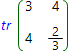# Matrix trace calculatorTrace of square matrix is the sum of diagonal elements of this matrix:

tr ( A ) = a1,1 + a2,2 + ... + ai,i + ... + an,n

This calculator finds trace of square matrix with step by step solution. As matrix elements one can input not only numbers and fractions but also the parameters.

Matrix trace calculatorA =